Function Repository Resource:

# IntegralApproximationPlot3D

Compute and plot an approximation to the integral of a function of two variables over a rectangle

Contributed by: Dennis M Schneider
 ResourceFunction["IntegralApproximationPlot3D"][f,{x,xmin,xmax},{y,ymin,ymax},method,{m,n}] plots f and an approximation to the integral of f over the rectangle determined by xmin≤x≤xmax and ymin≤y≤ymax based on the approximation method and regular partitions of the x- and y-axes with m and n equal subintervals. ResourceFunction["IntegralApproximationPlot3D"][f,{x,xmin,xmax},{y,ymin,ymax},method,n] returns ResourceFunction["IntegralApproximationPlot3D"][f,{x,xmin,xmax},{y,ymin,ymax},method,{n,n}].

## Details and Options

Following standard mathematical usage, the possible values of method are:
 "Upper" the height of the approximating rectangle is determined by the maximum value of the function on each subinterval "Midpoint" the height of the approximating rectangle is determined by the value of the function at the midpoint of each subinterval "Lower" the height of the approximating rectangle is determined by the minimum value of the function on each subinterval
The partition specification {n,n} can be shortened to {n} or simply n.
"CuboidStyle" is an option for ResourceFunction["IntegralApproximationPlot3D"] that applies a style to the cuboids.
"DrawGraph" is an option for ResourceFunction["IntegralApproximationPlot3D"] that, if set to False, will suppress the graph and just return the approximation to the area of the region.
"DrawSurface" is an option for ResourceFunction["IntegralApproximationPlot3D"] that, if set to False, will suppress the graph of the surface.
"PrintDisplay" is an option for ResourceFunction["IntegralApproximationPlot3D"] that, if set to False, will suppress printing of the approximation.

## Examples

### Basic Examples (1)

A midpoint sum approximation of a function over a rectangular region determined by a 20×25 grid:

 In:=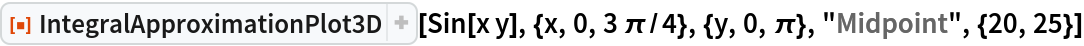Out=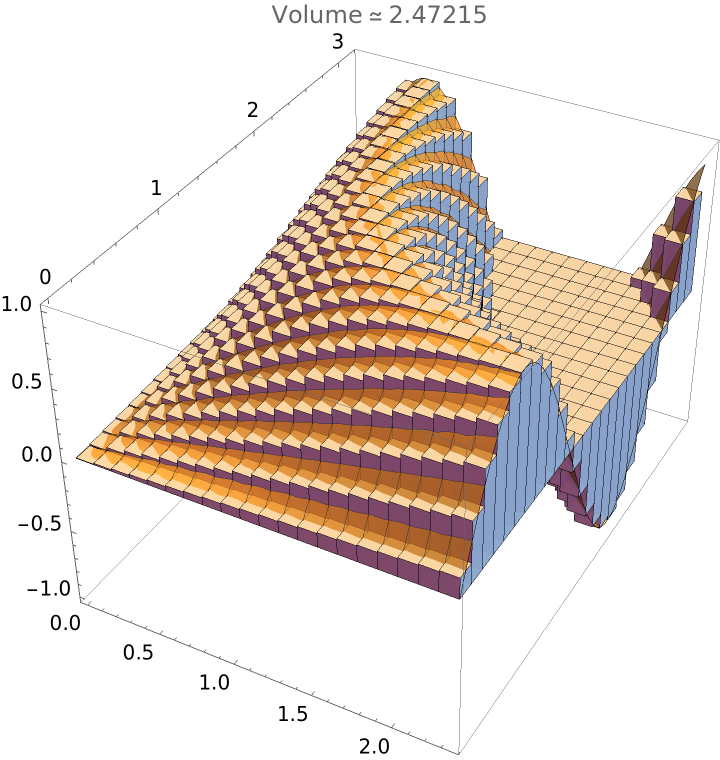### Scope (2)

A upper sum approximation over a square region. This will take a few seconds since there are 225 optimizations that must be performed:

 In:=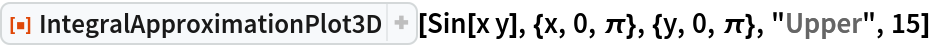Out=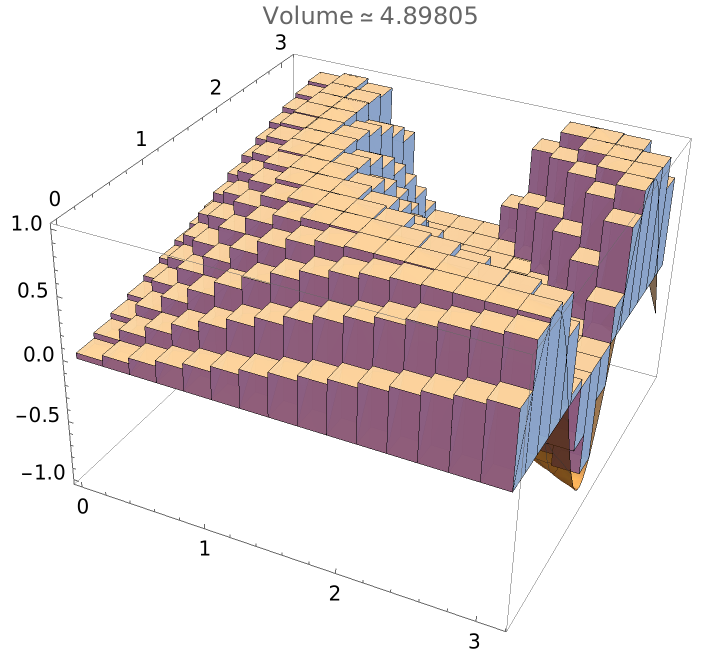A lower sum approximation with a rectangular partition. As with upper sum approximations, this will take a few seconds:

 In:=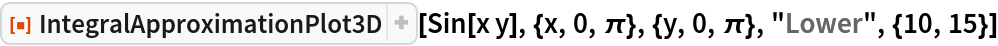Out=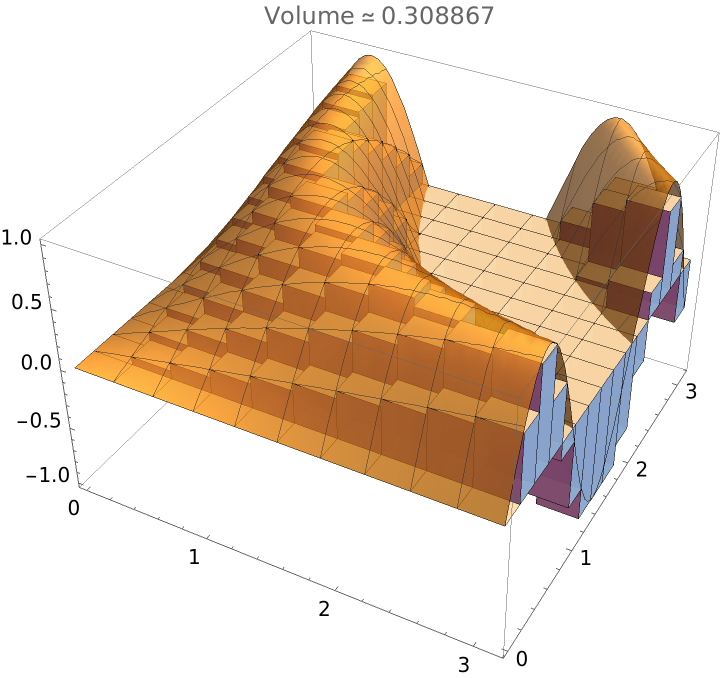### Options (3)

#### CuboidStyle (1)

Set a style for the cuboids to obtain a better view of the surface and change to a 15×15 grid:

 In:=Out=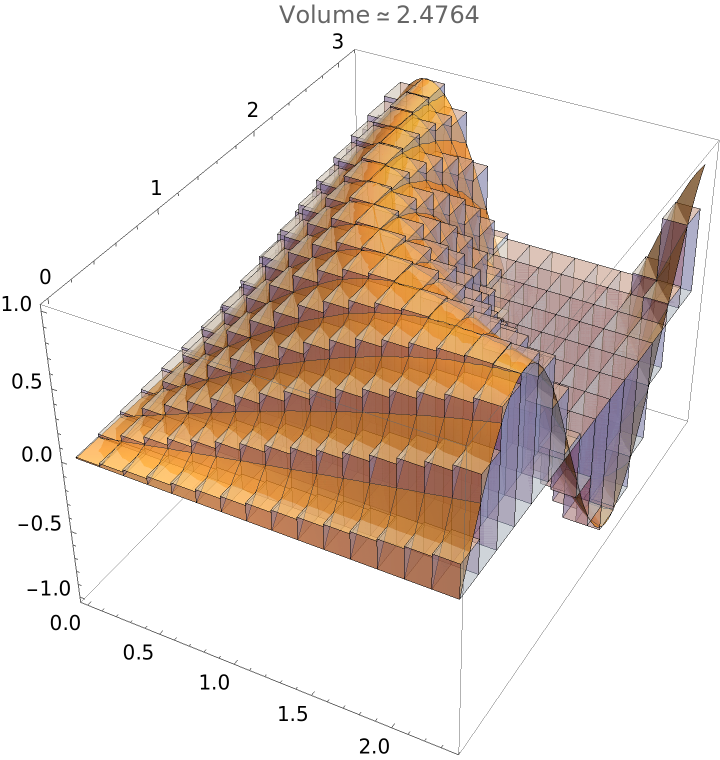#### DrawSurface (1)

Suppress the plot of the surface and the approximation of the volume and just show the cuboids:

 In:=Out=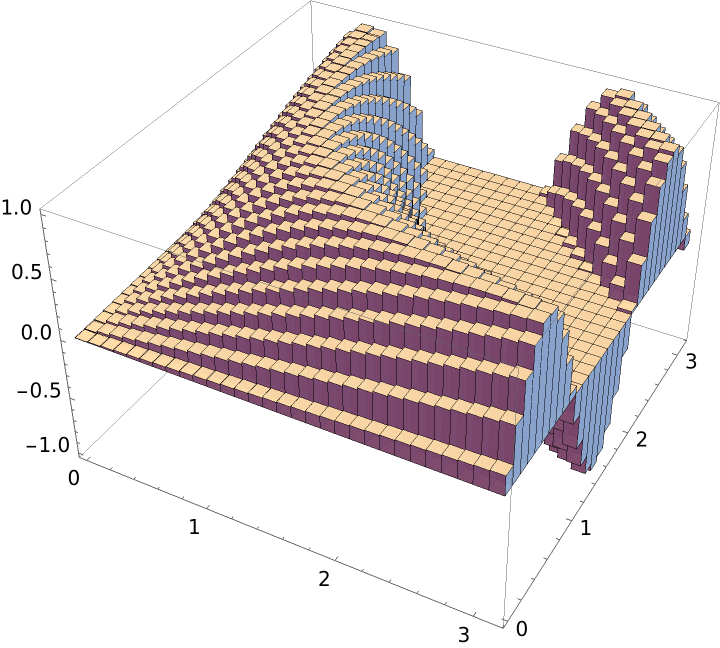#### DrawGraph (1)

Suppress the graphical output:

 In:=Out=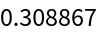## Publisher

Dennis M Schneider

## Version History

• 1.0.0 – 13 September 2019